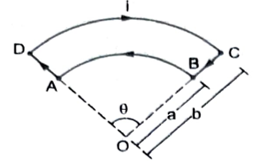Figure shows a current loop having two circular arcs joined by two radial lines. Find the magnetic field B at the centre O.

# Figure shows a current loop having two circular arcs joined by two radial lines. Find the magnetic field B at the centre O.1. A

$\frac{{\mathrm{\mu }}_{0}\mathrm{i\theta }\left(\mathrm{b}-\mathrm{a}\right)}{4\mathrm{\pi ab}}$

2. B

$\frac{2{\mathrm{\mu }}_{0}\mathrm{i\theta }\left(\mathrm{b}-\mathrm{a}\right)}{4\mathrm{\pi ab}}$

3. C

$\frac{3{\mathrm{\mu }}_{0}\mathrm{i\theta }\left(\mathrm{b}-\mathrm{a}\right)}{4\mathrm{\pi ab}}$

4. D

$\frac{5{\mathrm{\mu }}_{0}\mathrm{i\theta }\left(\mathrm{b}-\mathrm{a}\right)}{4\mathrm{\pi ab}}$

Register to Get Free Mock Test and Study Material

+91

Verify OTP Code (required)

### Solution:

As the point O is on the line AD, the magnetic field at O due to AD is zero. Similarly, the field at O due to BC is also zero. The field at the centre of a circular current loop is given  by $\mathrm{B}=\frac{{\mathrm{\mu }}_{0}\mathrm{i}}{2\mathrm{a}}$ . The field due to the circular arc BA will be ${\mathrm{B}}_{1}=\left(\frac{\mathrm{\theta }}{2\mathrm{\pi }}\right)\left(\frac{{\mathrm{\mu }}_{0}\mathrm{i}}{2\mathrm{a}}\right)$.
The right-hand thumb rule shows that the field is coming out of the plane of the figure. The field due to the circular arc DC is ${\mathrm{B}}_{2}=\left(\frac{\mathrm{\theta }}{2\mathrm{\pi }}\right)\left(\frac{{\mathrm{\mu }}_{0}\mathrm{i}}{2\mathrm{b}}\right)$ .
Going into the plane of the figure. The resultant field at O is $\mathrm{B}={\mathrm{B}}_{1}-{\mathrm{B}}_{2}=\frac{{\mathrm{\mu }}_{0}\mathrm{i\theta }\left(\mathrm{b}-\mathrm{a}\right)}{4\mathrm{\pi ab}}$
coming out of the plane.Register to Get Free Mock Test and Study Material

+91

Verify OTP Code (required)